Gauge your potential with Aakash BYJU'S JEE Main'23 Mock Test Gauge your potential with Aakash BYJU'S JEE Main'23 Mock Test

# JEE Main 2021 March 18 – Shift 1 Chemistry Question Paper with Solutions

Students can find the solutions of JEE Main 2021 March 18 Shift 1 Chemistry Paper on this page. These solutions have been prepared by expert teachers at BYJU’S. These JEE Main 2021 March 18 Shift 1 Chemistry Paper solutions will help them to understand the easy methods to solve the problems. Students can download the PDF format for offline use. Solving these questions will help students to improve their scores for the upcoming JEE Main exams.

### JEE Main 2021 March 18th Shift 1 Chemistry Question Paper

SECTION-A

Question 1. The ionic radius of Na+ ion is 1.02 Å. The ionic radii (in Å) of Mg2+ and Al3+, respectively are:

1. a. 0.72 and 0.54
2. b. 0.68 and 0.72
3. c. 1.05 and 0.99
4. d. 0.85 and 0.99

Solution:

For iso-electronic system,

$$r \alpha = \frac{1}{z_{effective}}$$Question 2. Match List-I with List-II:

 List-I List-II (Chemicals) (Use/Preparation/Constituent) (a) Alcoholic potassium hydroxide (i) electrodes in batteries (b) Pd/BaSO4 (ii) obtained by addition reaction (c) BHC (Benzene hexachloride) (iii) used for β-elimination reaction (d) Polyacetylene (iv) Lindlar’s Catalyst

Choose the most appropriate match:

1. a. (a)-(ii), (b)-(i), (c)-(iv), (d)-(iii)
2. b. (a)-(iii), (b)-(i), (c)-(iv), (d)-(ii)
3. c. (a)-(ii), (b)-(iv), (c)-(i), (d)-(iii)
4. d. (a)-(iii), (b)-(iv), (c)-(ii), (d)-(i)

Solution:

Alcoholic KOH ⇒ Used for beta - elimination reaction

Pd/ BaSO4 ⇒ Lindlar’s catalyst

BHC (Benzene hexachloride) ⇒ Addition product of benzene and

chlorine.

Question 3. The statements that are TRUE:

(A) methane leads to both global warming and photochemical smog

(B) methane is generated from paddy fields

(C) methane is a stronger global warming gas than CO2

(D) methane is a part of reducing smog.

Choose the most appropriate answer from the option given below:

1. a. (B), (C), (D) only
2. b. (A), (B), (C) only
3. c. (A), (B), (D) only
4. d. (A) and (B) only

Solution:

Contribution of global warming gas

CO2 > CH4 > CFC > O3 > N2O > H2O

But CH4 is 40 times stronger greenhouse gases than CO2. It has more heating effects.

Question 4. Compound with molecular formula C3H6O can show:

1. a. Both positional isomerism and metamerism
2. b. Metamerism
3. c. Positional isomerism
4. d. Functional group isomerism

Solution:

C3H6O DOU = 1

CH3 – CH2 – CH = O & CH3 – CO – CH3 are functional isomer. Therefore, functional group isomerism is the most appropriate.

Question 5. Match List-I with List-II:

 List-I List-II (a) Ca(OCl)2 (i) Antacid (b) CaSO4· 1/2H2O (ii) Cement (c) CaO (iii) Bleach (d) CaCO3 (iv) Plaster of Paris

Choose the most appropriate answer from the option given below:

1. a. (a)-(iii), (b)-(iv), (c)-(ii), (d)-(i)
2. b. (a)-(iii), (b)-(ii), (c)-(iv), (d)-(i)
3. c. (a)-(iii), (b)-(ii), (c)-(i), (d)-(iv)
4. d. (a)-(i), (b)-(iv), (c)-(iii), (d)-(ii)

Solution:

Ca(OCl)2 → Bleaching powder

CaSO4. 1/2H2O → Plaster of Paris

CaO → Cement

CaCO3 → Antacid

Question 6. In a binary compound, atoms of element A form a hcp structure and those of element M occupy 2/3 of the tetrahedral voids of the hcp structure. The formula of the binary compound is:

1. a. M2A3
2. b. MA3
3. c. M4A
4. d. M4A3

Solution:

A → hcp

M → 2/3rd of tetrahedral

M2 × 12/3 A6 = M8A6 = M4A3

Question 7. Match List-I with List-II:

 List-I List-II (Class of Drug) (Example) (a) Antacid (i) Novestrol (b) Artificial Sweetener (ii) Cimetidine (c) Antifertility (iii) Valium (d) Tranquilizers (iv) Alitame

Choose the most appropriate answer from the option given below:

1. a. (a)-(iv), (b)-(iii), (c)-(i), (d)-(ii)
2. b. (a)-(ii), (b)-(iv), (c)-(i), (d)-(iii)
3. c. (a)-(ii), (b)-(iv), (c)-(iii), (d)-(i)
4. d. (a)-(iv), (b)-(i), (c)-(ii), (d)-(iii)

Solution:

Antacid — Cimetidine

Artificial sweetener — Alitame

Antifertility — Novestrol

Tranquillizers — Valium

Question 8. Reagent, 1-naphthylamine and sulfanilic acid in acetic acid is used for the detection of:

1. a. NO
2. b. N2O
3. c. NO-3
4. d. NO-2

Solution:

For detection of NO2-, the following test is used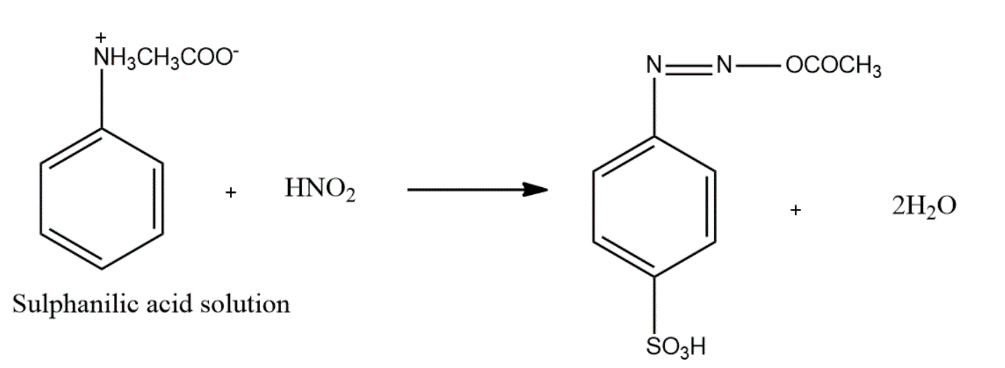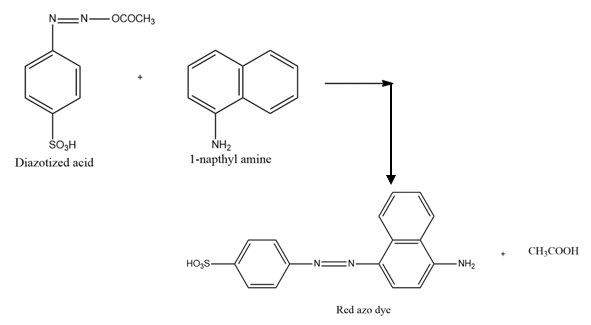Question 9. The correct structures of trans-[NiBr2(PPh3)2] and meridional-[Co(NH3)3(NO2)3] respectively are: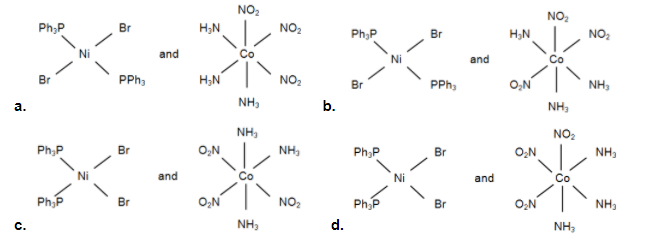Solution: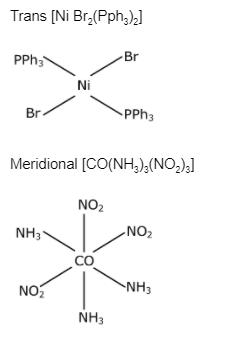Question 10. Match List-I with List-II:

 List-I List-II (a) Chlorophyll (i) Ruthenium (b) Vitamin – B12 (ii) Platinum (c) Anticancer drug (iii) Cobalt (d) Grubbs catalyst (iv) Magnesium

Choose the most appropriate answer from the option given below:

1. a. (a)-(iv), (b)-(iii), (c)-(i), (d)-(ii)
2. b. (a)-(iii), (b)-(ii), (c)-(iv), (d)-(i)
3. c. (a)-(iv), (b)-(iii), (c)-(ii), (d)-(i)
4. d. (a)-(iv), (b)-(ii), (c)-(iii), (d)-(i)

Solution:

⇒ Cis – Platin [Pt (NH3)2Cl2] used in the treatment of cancer

⇒ Chlorophyll is complex of Mg

⇒ Vitamin B12 is a complex of CO

⇒ Grubbs catalyst is a series of catalysts containing ruthenium

Question 11. The number of ionisable hydrogens present in the product obtained from a reaction of phosphorus trichloride and phosphonic acid is:

1. a. 3
2. b. 1
3. c. 0
4. d. 2

Solution:

PCl3 + H3PO3 +H2O → H4P2O5 + HCl

pyrophosphorous acid

The structure of pyrophosphorous acid shows that it has two acidic or ionisable hydrogen.

Question 12. A certain orbital has no angular nodes and two radial nodes. The orbital is:

1. a. 2p
2. b. 3p
3. c. 2s
4. d. 3s

Solution:

No angular nodes ⇒ ℓ = 0

Radial nodes = n – ℓ –1 = n – 0 – 1 = 2

n = 3

Question 13. Consider the above chemical reaction and identity product “A”: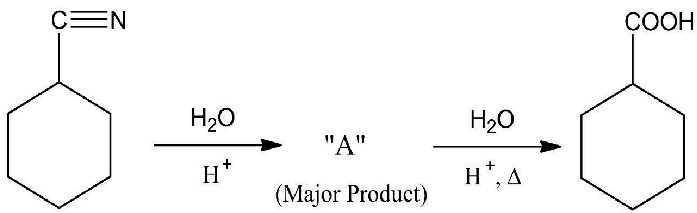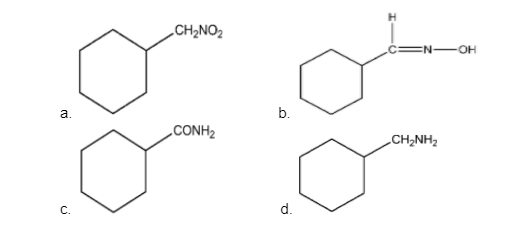Solution:Question 14. Given below are two statements: One is labelled as Assertion A and the other is labelled as Reason R:

Assertion A: During the boiling of water having a temporary hardness, Mg(HCO3)2 is converted to MgCO3.

Reason R: The solubility product of Mg(OH)2 is greater than that of MgCO3.

In the light of the above statements, choose the most appropriate answer from the options given below.

1. a. A is false but R is true
2. b. Both A and R are true and R is the correct explanation of A
3. c. Both A and R are true but R is NOT the correct explanation of A
4. d. A is true but R is false

Solution:

Mg(HCO3)2 → Mg(OH)2 ↓ + CO2

Temporary Hardness

Ca(HCO3)2 → CaCO3 ↓ + CO2 + H2O

Ksp Mg(OH)2 > Ksp MgCO3

And hence, Mg(OH)2 precipitation first.

Question 15. The chemical is added to reduce the melting point of the reaction mixture during the extraction of aluminium is:

1. a. Cryolite
2. b. Calamine
3. c. Kaolite
4. d. Bauxite

Solution:

For reducing the melting point of Alumina, Cryolite i.e. Na3AlF6 is added.

Question 16. Considering the below chemical reaction, identity the product “X”: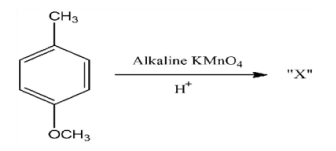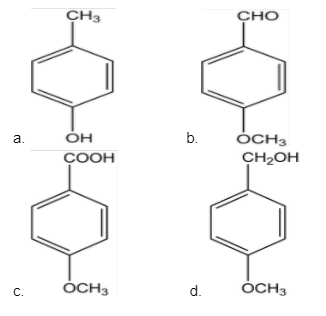Solution: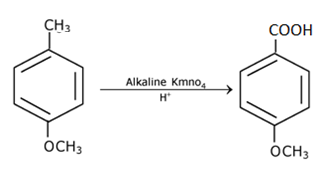Question 17. Considering the below reaction, X and Y respectively are: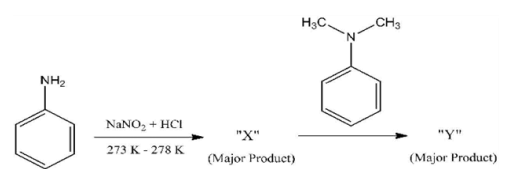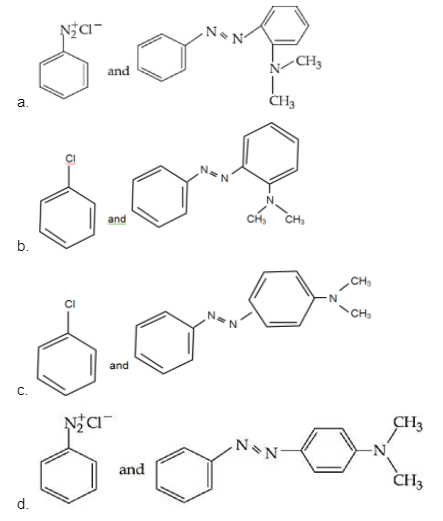Solution: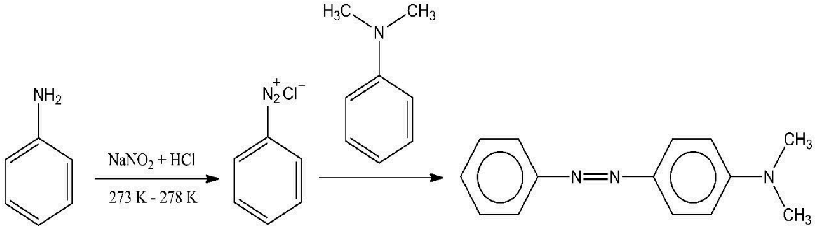Question 18. Reaction of Grignard reagent, C2H5MgBr with C8H8O followed by hydrolysis gives compound “A” which reacts instantly with Lucas reagent to give compound B, C10H13Cl. The Compound B is: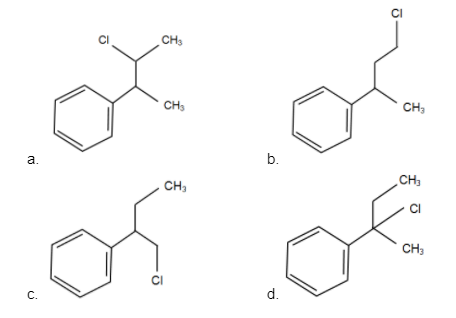Solution:

$$C_{8}H_{8}O\xrightarrow[H+]{C_{2}H_{5}mgBr}(A)\overset{Lucas \: reagent}{\rightarrow}(B)C_{10}H_{13}Cl$$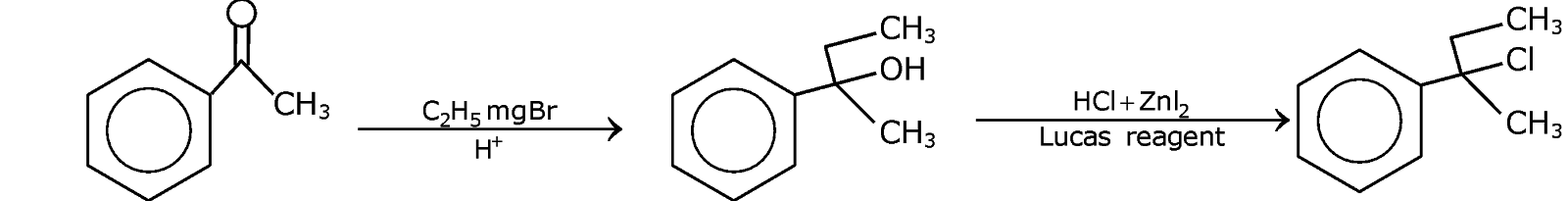Question 19. A non-reducing sugar ”A” hydrolyses to give two reducing monosaccharides. Sugar A is:

1. a. Glucose
2. b. Fructose
3. c. Sucrose
4. d. Galactose

Solution:

C12H22O11 + H2O → C6H12O6 + C6H12O6

Sucrose D-glucose D-fructose

(Non-reducing sugar) (reducing sugar)

A non-reducing sugar is a carbohydrate that is not oxidized by a weak oxidizing agent (an oxidizing agent that oxidises aldehydes but not alcohols, such as the Tollen’s reagent) in a basic aqueous solution.

Question 20. Match List-I with List-II:

 List-I List-II (Process) (Catalyst) (a) Deacon’s process (i) ZSM-5 (b) Contact process (ii) CuCl2 (c) Cracking of hydrocarbons (iii) iParticles ’Ni’ (d) Hydrogenation of vegetable oils (iv) V2O5

Choose the most appropriate answer from the option given below:

1. a. (a)-(i), (b)-(iii), (c)-(ii), (d)-(iv)
2. b. (a)-(iv), (b)-(ii), (c)-(i), (d)-(iii)
3. c. (a)-(ii), (b)-(iv), (c)-(i), (d)-(iii)
4. d. (a)-(iii), (b)-(i), (c)-(iv), (d)-(ii)

Solution:

Deacon's process is used for industrial preparation of Chlorine gas

$$HCl + O_{2} \overset{CuCl_{2}}{\rightarrow} H_{2}O + Cl_{2}$$

Contact process is used for industrial preparation of sulphuric acid & V2O5 in catalyst involved in the process.$$CxH_{4} \overset{ZSM-5\: Cracking}{\rightarrow} products$$

SECTION - B

Question 21. 2 molal solution of a weak acid HA has a freezing point of 3.885 oC. The degree of dissociation of this acid is _________ × 10–3. (Round off to the Nearest Integer). [Given: Molal depression constant of water = 1.85 K kg mol–1Freezing point of pure water = 00C]

Solution:

Tf sol. = – 3.885°C

ΔTf = +3.885 = i × kf × m

3.885 = i × 1.85 × 2

$$i = \frac{3.885}{1.85\times 2} = [1+\alpha ]$$

$$\alpha = \frac{0.185}{3.7} = 0.05 = 50\times 10^{-3}$$

Question 22. The total number of unpaired electrons present in the complex K3[Cr(oxalate)3] is _______.

Solution:

K3[Cr(ox)3]

Chromium & in + 3 oxidation state

Cr → 3d5 4s1

Cr3+ → 3d3 3 unpaired electron the hybridization of chromium in the complex is d2sp3

Question 23. AX is a covalent diatomic molecule where A and X are second-row elements of the periodic table. Based on Molecular orbital theory, the bond order of AX is 2.5. The total number of electrons in AX is __________. (Round off to the Nearest Integer).

Solution:

The compound AX is NO; its bond order is 2.5 & it has a total of 15 electrons.

Question 24. ___________ grams of 3-Hydroxy propanal (MW = 74) must be dehydrated to produce 7.8 g of acrolein (MW = 56) (C3H4O) if the percentage yield is 64. (Round off to the Nearest Integer).

[Given: Atomic masses: C : 12.0 u, H : 1.0 u, O : 16.0 u]

Solution: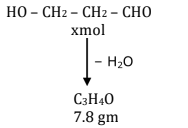7.8/ 56 = 0.14 mol

% yield =

$$\frac{7.8 / 56}{x}\times 100 = 64$$

$$x = \frac{7.8\times 100}{56\times 64} = \frac{780}{56\times 64}mol$$

$$W_{reactant} = \frac{780}{56\times 64}\times 74 = 16.11 g$$

Question 25. A reaction of 0.1 mole of Benzyl amine with bromomethane gave 23 g of Benzyl trimethyl ammonium bromide. The number of moles of bromomethane consumed in this reaction are n × 10–1, when n = ___________ . (Round off to the Nearest Integer). [Given: Atomic masses: C: 12.0 u, H : 1.0 u, N : 14.0 u, Br : 80.0 u]

Solution:

Ph – CH2NH2 + 3CH3Br → PhCH2N+(Me)3Br-

0.1 mol 23 / 230 = 0.1 mol

∴ moles of CH3Br = 0.3 = 3 × 10–1 mol

Question 26. 2NO (g) + Cl2 (g) → 2 NOCl (s): This reaction was studied at – 100C and the following data was obtained.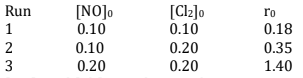[NO]0 and [Cl2]0 are the initial concentrations and r0 is the initial reaction rate. The overall order of the reaction is _________. (Round off to the Nearest Integer).

Solution:

Exp. (I) 0.18 = K (0.1) x (0.1) +y .... (1)

Exp. (II) 0.35 = K (0.1) x (0.2) y .... (2)

Exp. (III) 1.40 = K (0.2) x(0.2)y .... (3)

(2) ÷ (3)

$$\frac{0.35}{1.40}=\frac{K\times(0.1)^{x}(0.2)^{y}}{K(0.2)^{x}(0.2)^{y}}$$

$$\frac{1}{4} = \left ( \frac{1}{2} \right )^{x} \Rightarrow x = 2$$

(1) ÷ (2)

$$\frac{1}{2} = \left ( \frac{1}{2} \right )^{y} \Rightarrow y = 1$$

Question 27. For the reaction: 2Fe3+ (aq) + 2I (aq) → 2Fe2+ (aq) + I2(s)

The magnitude of the standard molar free energy change, ΔrGom = – _______ kJ (Round off the Nearest Integer).Solution:

2Fe3+(aq) + 2I(aq) → 2Fe+2(aq) + I2(s)

$$1\times E^{o}_{Fe^{+3}/Fe^{+2}}+2\times E^{o}_{Fe^{+2}/Fe} = 3\times E^{o}_{Fe^{+3}/Fe}$$

$$E^{o}_{Fe^{+3}/Fe^{+2}}$$
= 3 × (–0.036) – 2 × (–0.44)

= – 0.108 + 0.

= 0.772 V

$$E^{o}_{cell} = E^{o}_{Fe^{+3}/Fe^{+2}}+ E^{o}_{I^{-}/I_{2}}$$

= 0.772 – 0.539 = 0.233 V

ΔG° nF Eocell

= +2 × 96500 × 0.233

= 44969 J = 44.9 KJ ≃ 45 KJ

Question 28. For the reaction: C2H6→ C2H4 + H2 : The reaction enthalpy ΔrH = __________ kJ mol–1. (Round off to the Nearest Integer).

[Given: Bond enthalpies in kJ mol–1; C – C: 347, C = C : 611; C – H : 414; H – H ; 436]

Solution:

C2H6 → C2H4 + H2

ΔH = ??

ΔH = [ (E c-c + 6 E C-H) – (E C=C + 4E C-H + E H-H)

ΔH= [ 347 + 6*414] –(611+ 4*414 + 433] = 2831 - 2700

= 131 kJ/mol

Question 29. In order to prepare a buffer solution of pH 5.74, sodium acetate is added to acetic acid. If the concentration of acetic acid in the buffer is 1.0 M, the concentration of sodium acetate in the buffer is _________ M. (Round off to the Nearest Integer).

[Given: pKa (acetic acid) = 4.74]

Solution:

Buffer pH = 5.74 = P(Ka) (acetic acid) + log sodium acetate / acetic acid

So, on solving, sodium acetate / acetic acid = 10

Sodium acetate = 10 M

Question 30. Complete combustion of 3 g of ethane gives x × 1022 molecules of water. The value of x is ________. [Round off to the Nearest Integer].

[Use: NA = 6.023 × 1023; Atomic masses in u : C : 12.0; O : 16.0 : H : 1.0 ]

Given: 18

Solution:

C2H6 + O2 → 2CO2 + 3H2O

3gm 0.3 mol

0.1 mol 0.3 NA

= 0.3 × 6.023 × 1023 molecules of H2O

= 1.8069 × 1023

= 18.069 × 1022

### JEE Main 2021 Chemistry Paper March 18 Shift 1